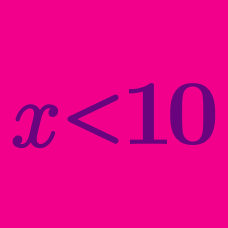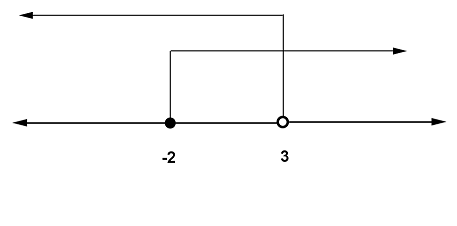Algebra

# Two-sided Linear Inequalities

What is the greatest integer value of $x$ that satisfies the inequality $-5 \leq 2x - 3 < 3?$

Solve the simultaneous inequalities

\begin{aligned} -3x + 4 &\ge 1 \\ 2x - 5 &< 5x + 1. \end{aligned}

Solve the inequalities $\dfrac{x-2}{3} < \dfrac{x+5}{4} < \dfrac{x-1}{2}.$

The minimum value of $x$ that satisfies the inequality $\dfrac{x - 5}{11 x - 4} \geq 3$ can be expressed as $\dfrac{a}{b}$, where $a$ and $b$ are coprime positive integers. What is the value of $\dfrac{a}{b}?$If the range of $x$ represented by the arrows in the number line above is equivalent to $x - a < -x - 1 \le x + b,$ what is $a + b ?$

×